#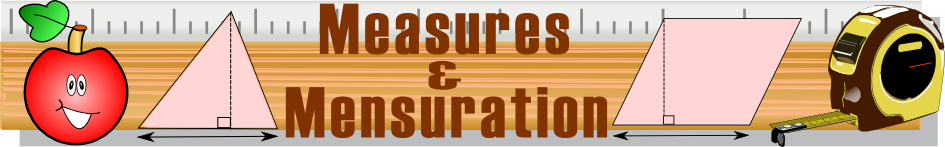There are 366 different Starters of The Day, many to choose from. You will find below some starters on the topic of Mensuration. A lesson starter does not have to be on the same topic as the main part of the lesson or the topic of the previous lesson. It is often very useful to revise or explore other concepts by using a starter based on a totally different area of Mathematics.

Main Page

### Mensuration Starters: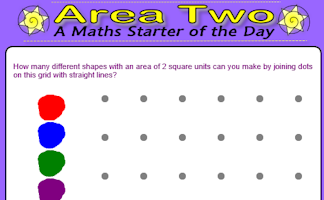How many different shapes with an area of 2 square units can you make by joining dots on this grid with straight lines?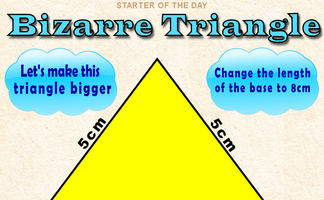By how much would the area of this triangle increase if its base was enlarged to 8cm?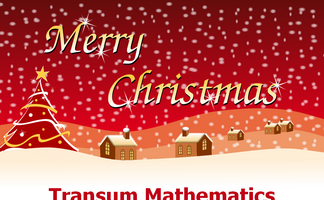Which of the two shapes has the largest area? You will be surprised!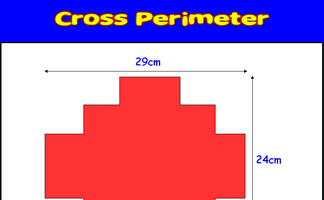Calculate the distance around the given shapeFind the loci of the goat's position as it eats the grass while tethered to the rope.Introduce linear equations by solving these problems about lengths.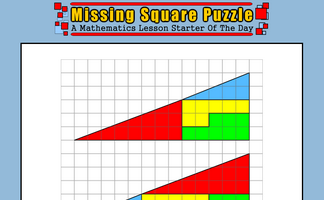The missing square puzzle is an optical illusion used to help students reason about geometrical figures.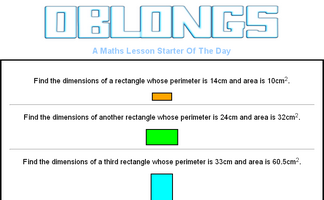Find the dimensions of a rectangle given the perimeter and area.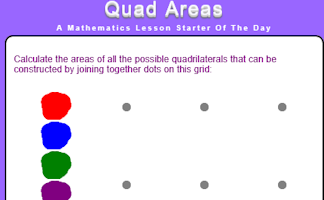Calculate the areas of all the possible quadrilaterals that can be constructed by joining together dots on this grid.A quick quiz about five items on a shopping list written 40 years ago.Use the information implied in the diagram to calculate the perimeter of this shape.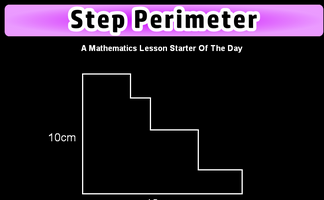Is it possible to work out the perimeter of this shape if not all the side lengths are given?

## Exercises#### Algebraic Perimeters

Questions about the perimeters and areas of polygons given as algebraic expressions.#### Area and Perimeter

Show that you know the area and perimeter formulas of basic shapes.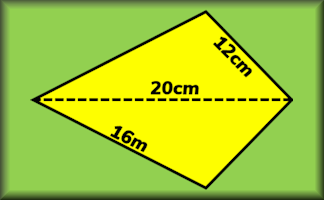#### Area and Perimeter of a Kite

A short exercise to practise using the formulae for area and perimeter of a kite.#### Area and Perimeter of a Parallelogram

Many different ways to practise your skills finding the areas and perimeters of parallelograms.#### Area and Perimeter of a Rectangle

Questions on the areas and perimeters of rectangles which will test your problem solving abilities.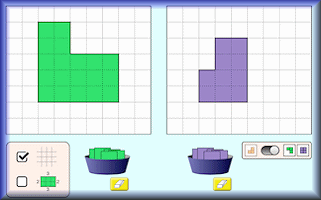#### Area Builder

An interactive workspace in which to make shapes using square tiles with given areas and perimeters.#### Area Maze

Use your knowledge of rectangle areas to calculate the missing measurement of these composite diagrams.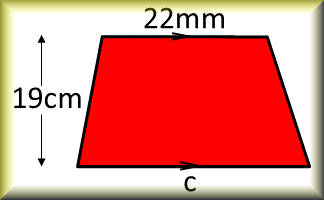#### Area of a Trapezium

Check that you can find the area of a trapezium and use the trapezium area formula for problem solving.#### Area of a Triangle

Calculate the areas of the given triangles in this self marking quiz.#### Area Two

How many different shapes with an area of 2 square units can you make by joining dots on this grid with straight lines?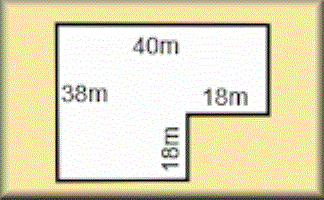#### Areas of Composite Shapes

Find the areas of combined (composite) shapes made up of one or more simple polygons and circles.#### Bottles, Boxes and Cans

Estimate the capacity of the bottles, boxes and cans in the photograph and answer questions about volume.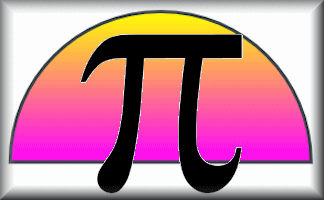#### Circles

Practise using pi to calculate various circle measurements. There are six levels of difficulty.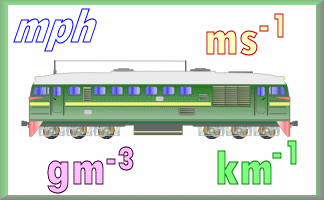#### Compound Units

Practise using compound units such as speed, unit pricing and density to solve problems.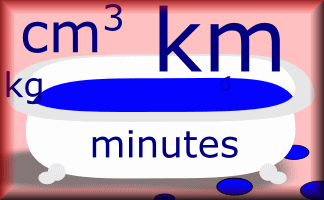#### Converting Standard Units

Converting measurements of length, mass, volume and time from one unit of measure to another.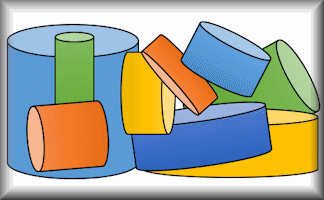#### Cylinders

Apply formulae for the volumes and surface areas of cylinders to answer a wide variety of questions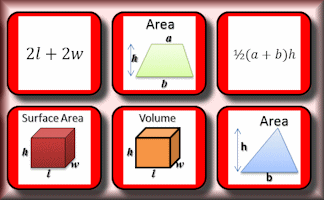#### Formulae Pairs

Find the matching pairs of diagrams and formulae for basic geometrical shapes.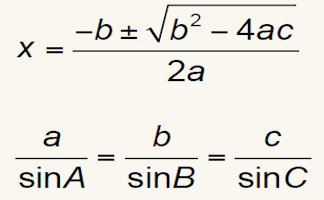#### Formulae to Remember

The traditional pairs or pelmanism game adapted to test recognition for formulae required to be memorised for GCSE exams.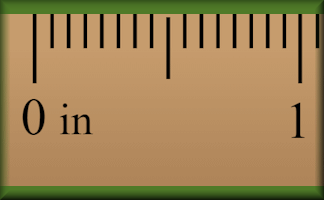#### Imperial Units

Learn about common imperial units and how they relate to other units of measurement.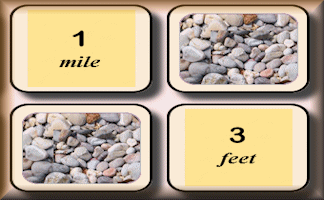#### Imperial Units Pairs

Find the matching pairs of equivalent imperial units in this interactive online game.#### Inequalities

Check that you know what inequality signs mean and how they are used to compare two quantities. Includes negative numbers, decimals, fractions and metric measures.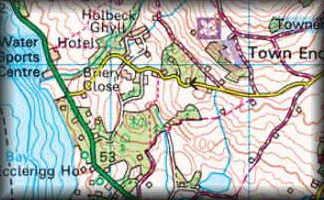#### Map Scales

Test your understanding of map scales expressed as ratios with this self marking quiz.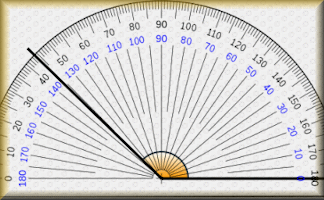#### Measuring Angles

Measure the size of the given angles to within two degrees of their actual value.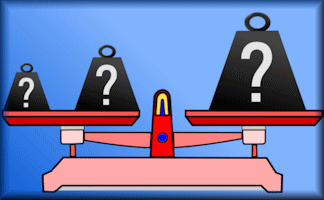#### Measuring Units

Check your knowledge of the units used for measuring with this self-marking quiz about metric and imperial units.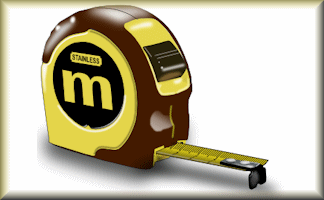#### Memorable Measures

This is a visual aid and printable cards to introduce a homework activity about measures.#### Metric Units Pairs

Find the matching pairs of equivalent metric units in this interactive online game.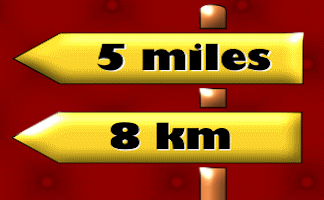#### Mileometer

Practise converting between miles and kilometres with this self marking quiz.#### Pin Board

Rows and columns of dots that can be joined using straight lines to create shapes.Calculate the areas of all the possible quadrilaterals that can be constructed by joining together dots on this grid.A self marking exercise on the reading of scales of different types.#### Scale Drawings

Measure line segments and angles in geometric figures, including interpreting scale drawings.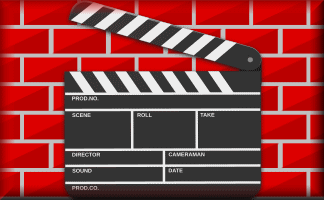#### Screen Test

Memorise the mathematical facts in the video then answer the ten quiz questions.#### Sorting Units

Order the ten containers according to their value (money, length and weight)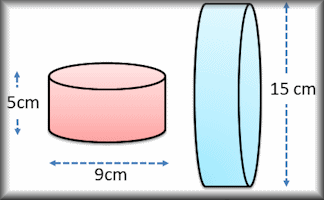#### Surface Area

Work out the surface areas of common solid shapes in this collection of exercises.#### Volume

Use formulae to solve problems involving the volumes of cuboids, cones, pyramids, prisms and composite solids.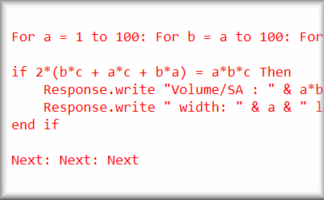#### Volume Equals Surface Area

Find the cuboids with integer side lengths where the volume is numerically equal to the surface area.

### Search

The activity you are looking for may have been classified in a different way from the way you were expecting. You can search the whole of Transum Maths by using the box below.

Have today's Starter of the Day as your default homepage. Copy the URL below then select
Tools > Internet Options (Internet Explorer) then paste the URL into the homepage field.

Set as your homepage (if you are using Internet Explorer)

Do you have any comments? It is always useful to receive feedback and helps make this free resource even more useful for those learning Mathematics anywhere in the world. Click here to enter your comments.For All: